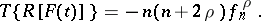# Gegenbauer transform

The integral transformof a function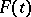,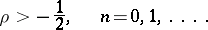Hereare the Gegenbauer polynomials. If a function can be expanded into a generalized Fourier series by Gegenbauer polynomials, the following inversion formula is valid: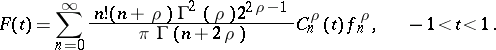The Gegenbauer transform reduces the differentiation operation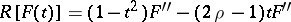to the algebraic operation